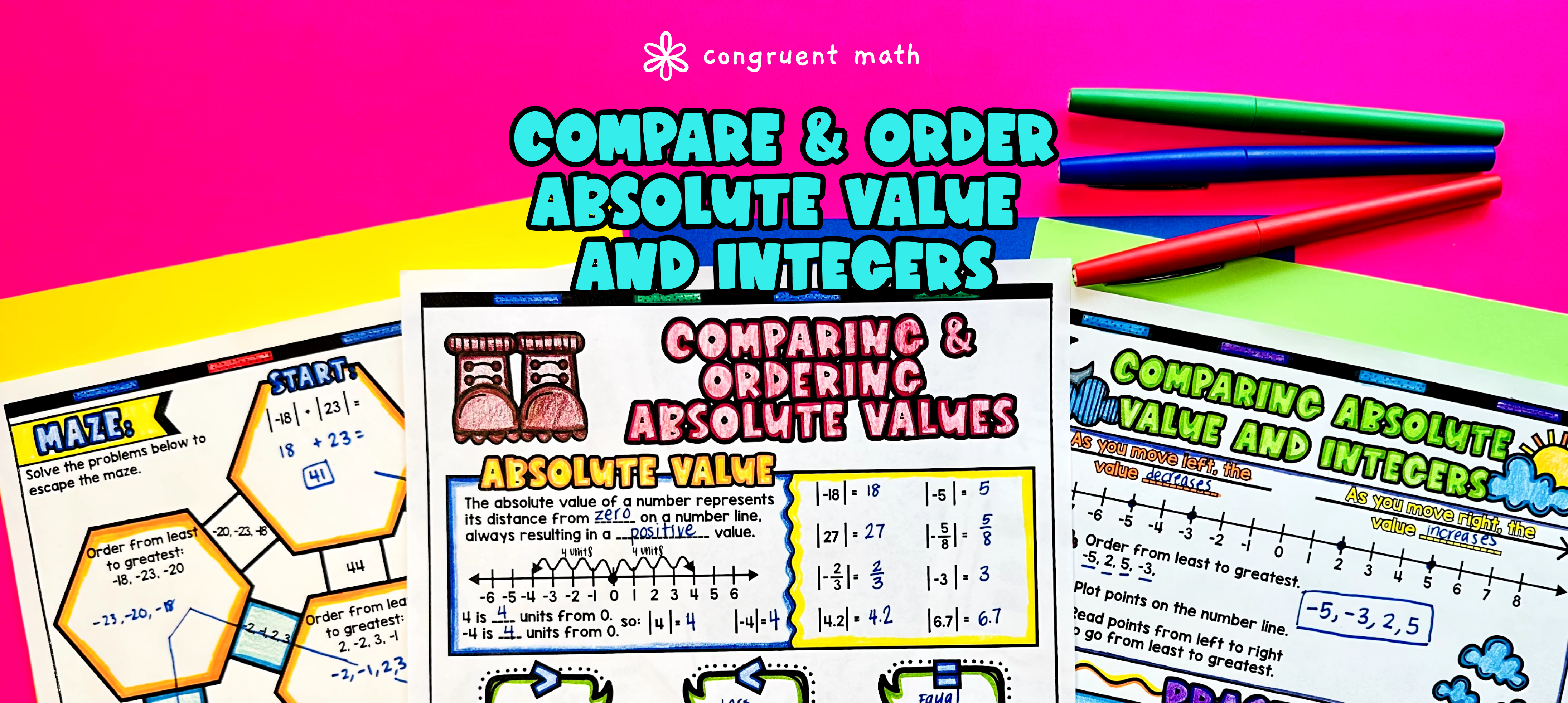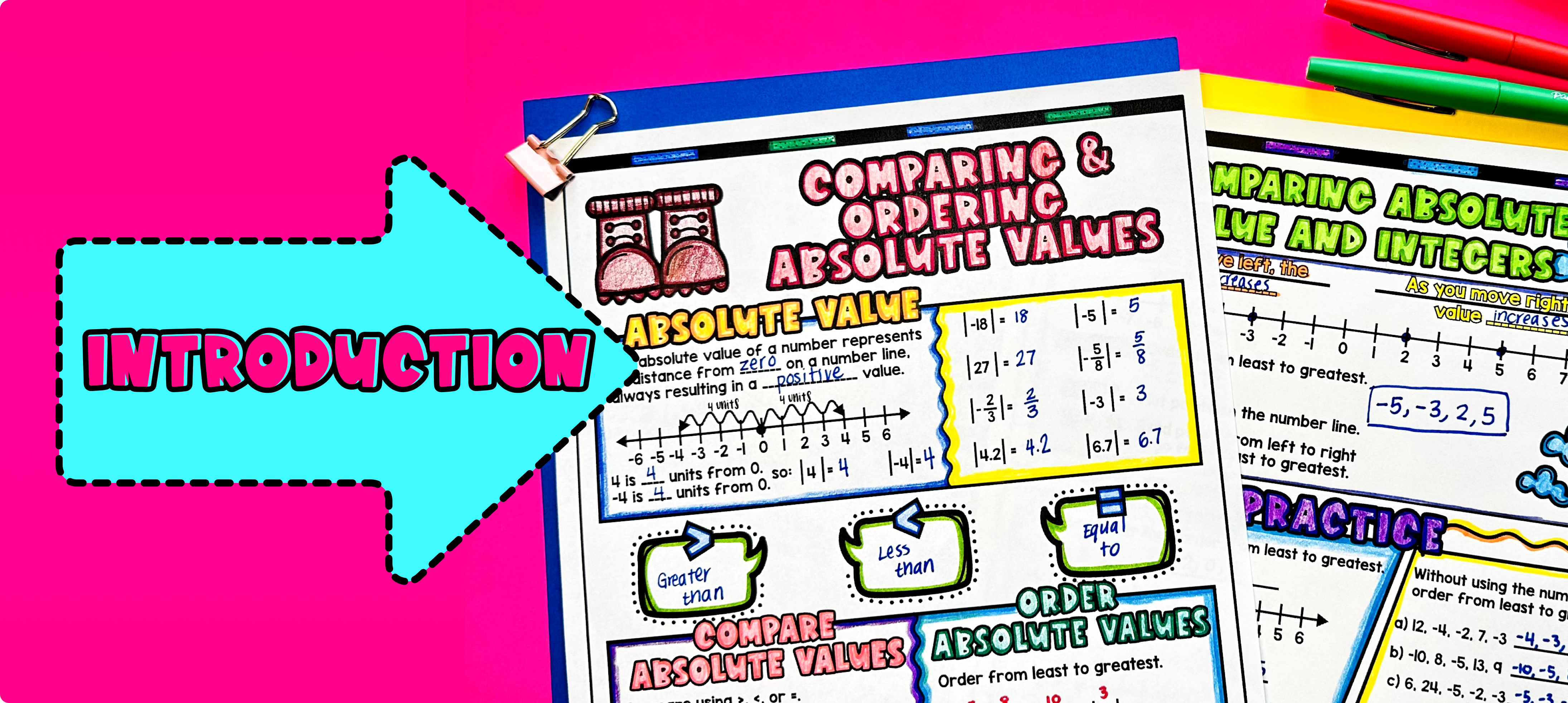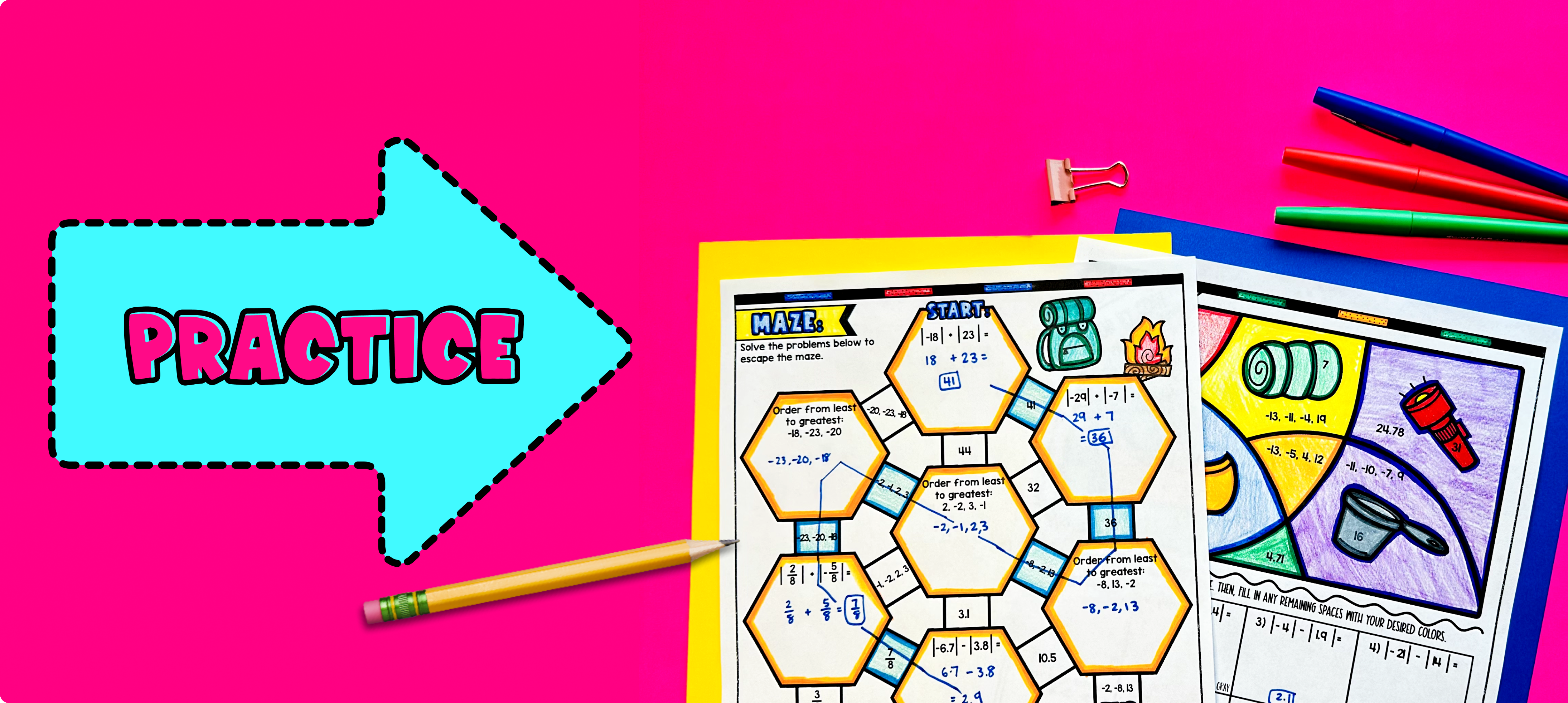# Comparing and Ordering Integers & Absolute Values of Rational Numbers Lesson PlanPin This

## Overview

Ever wondered how to teach comparing and ordering integers and the absolute values of rational numbers in an engaging way to your 6th grade math students?

In this lesson plan, students will learn about comparing and ordering integers, absolute values of integers and rational numbers, and their real-life applications. Through artistic, interactive guided notes, check for understanding questions, a doodle and color by number activity, and a maze worksheet, students will gain a comprehensive understanding of comparing and ordering integers and absolute values of rational numbers.

The lesson culminates with a real-life example that explores how these concepts can be applied to grocery shopping. Students will have the opportunity to read and write about adding and subtracting decimals as it relates to making purchases at the grocery store.

## Get the Lesson Materials\$4.25

## Learning Objectives

After this lesson, students will be able to:

• Define and explain absolute value of rational numbers

• Compare and order integers using number line diagrams

• Apply the concept of integers and absolute value to real-life situations

• Solve problems involving comparing and ordering integers and rational numbers.

## Prerequisites

Before this lesson, students should be familiar with:

• Understanding of the number line and the concept of positive and negative numbers

## Materials

• Pencils

• Colored pencils or markers

• Comparing and Ordering Integers & Absolute Values Guided notes

## Key Vocabulary

• Absolute value

• Rational numbers

• Integers

## Procedure

### IntroductionPin This

As a hook, ask students why it is important to understand the absolute value of rational numbers and how it can be useful in real-life situations. Refer to the last page of the guided notes as well as the FAQs below for ideas.

Use the first page of the guided notes to introduce the concept of absolute value of rational numbers. Explain that the absolute value of a number is its distance from zero on a number line, and it is always positive or zero. Walk through the key points of the topic, such as how to find the absolute value of a rational number and how to compare the values. Explain the concept of comparing rational numbers using the greater than (>), less than (<), and equal to (=) symbols.

Use the second page of the guided notes to introduce comparing and ordering integers using number lines. Walk through the key points of the topic, such as how to plot integers with different signs and how to order them from least to greatest or vice versa.

Based on student responses, reteach concepts that students need extra help with. If your class has a wide range of proficiency levels, you can pull out students for reteaching, and have more advanced students begin work on the practice exercises.

### PracticePin This

Have students practice comparing and ordering integers and absolute values of rational numbers using the maze and color by code activity. Walk around the classroom to answer any questions students may have.

### Real-Life ApplicationPin This

Bring the class back together, and introduce the concept of comparing positive and negative integers as applied to representing elevations. Use the last page of the guided notes for this.

For example, Mount Everest is at 8,846 meters above sea level, while the lowest elevation in the United States, found in Death Valley, California, is 86 meters below sea level, represented as -86.

Refer to the guided notes resource for more ideas on how to teach real-world applications of comparing and ordering integers and absolute values of rational numbers.

## Extensions

If you’re looking for digital practice for comparing and ordering integers and absolute values, try my Pixel Art activities in Google Sheets. Every answer is automatically checked, and correct answers unlock parts of a mystery picture. It’s incredibly fun, and a powerful tool for differentiation.

Here are 2 activities to explore: